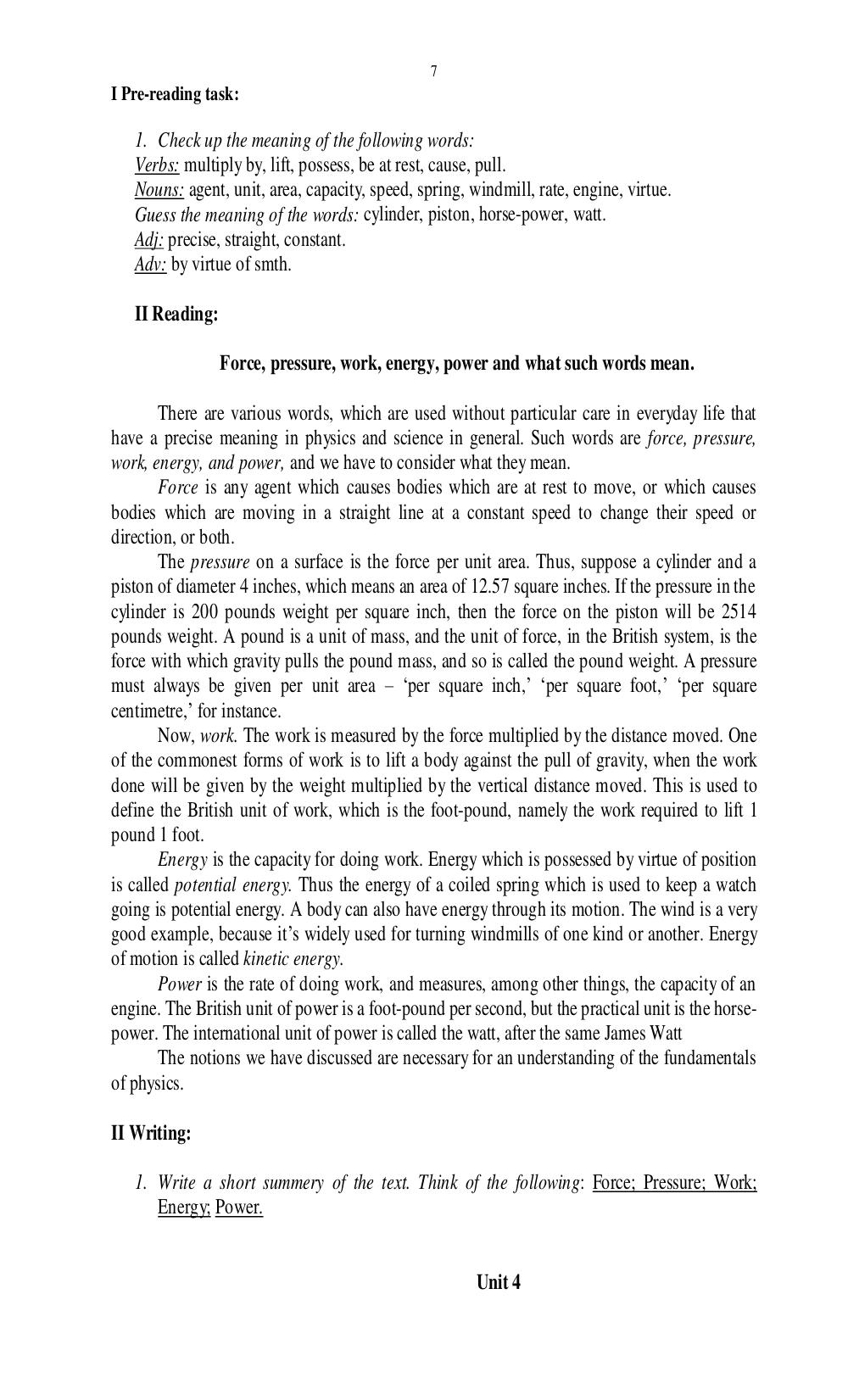# Some Properties of Matter. Грекова О.А. - 7 стр.

Составители:

Рубрика:

• ## Иностранный язык7
1. Check up the meaning of the following words:
Verbs: multiply by, lift, possess, be at rest, cause, pull.
Nouns: agent, unit, area, capacity, speed, spring, windmill, rate, engine, virtue.
Guess the meaning of the words:
cylinder, piston, horse-power, watt.
Force, pressure, work, energy, power and what such words mean.
There are various words, which are used without particular care in everyday life that
have a precise meaning in physics and science in general. Such words are force, pressure,
work, energy, and power, and we have to consider what they mean.
Force is any agent which causes bodies which are at rest to move, or which causes
bodies which are moving in a straight line at a constant speed to change their speed or
direction, or both.
The pressure on a surface is the force per unit area. Thus, suppose a cylinder and a
piston of diameter 4 inches, which means an area of 12.57 square inches. If the pressure in the
cylinder is 200 pounds weight per square inch, then the force on the piston will be 2514
pounds weight. A pound is a unit of mass, and the unit of force, in the British system, is the
force with which gravity pulls the pound mass, and so is called the pound weight. A pressure
must always be given per unit area per square inch, per square foot, per square
centimetre, for instance.
Now, work. The work is measured by the force multiplied by the distance moved. One
of the commonest forms of work is to lift a body against the pull of gravity, when the work
done will be given by the weight multiplied by the vertical distance moved. This is used to
define the British unit of work, which is the foot-pound, namely the work required to lift 1
pound 1 foot.
Energy is the capacity for doing work. Energy which is possessed by virtue of position
is called potential energy. Thus the energy of a coiled spring which is used to keep a watch
going is potential energy. A body can also have energy through its motion. The wind is a very
good example, because its widely used for turning windmills of one kind or another. Energy
of motion is called kinetic energy.
Power is the rate of doing work, and measures, among other things, the capacity of an
engine. The British unit of power is a foot-pound per second, but the practical unit is the horse-
power. The international unit of power is called the watt, after the same James Watt
The notions we have discussed are necessary for an understanding of the fundamentals
of physics.
II Writing:
1. Write a short summery of the text. Think of the following: Force; Pressure; Work;
Energy; Power.
Unit 47

1. Check up the meaning of the following words:
Verbs: multiply by, lift, possess, be at rest, cause, pull.
Nouns: agent, unit, area, capacity, speed, spring, windmill, rate, engine, virtue.
Guess the meaning of the words: cylinder, piston, horse-power, watt.

Force, pressure, work, energy, power and what such words mean.

There are various words, which are used without particular care in everyday life that
have a precise meaning in physics and science in general. Such words are force, pressure,
work, energy, and power, and we have to consider what they mean.
Force is any agent which causes bodies which are at rest to move, or which causes
bodies which are moving in a straight line at a constant speed to change their speed or
direction, or both.
The pressure on a surface is the force per unit area. Thus, suppose a cylinder and a
piston of diameter 4 inches, which means an area of 12.57 square inches. If the pressure in the
cylinder is 200 pounds weight per square inch, then the force on the piston will be 2514
pounds weight. A pound is a unit of mass, and the unit of force, in the British system, is the
force with which gravity pulls the pound mass, and so is called the pound weight. A pressure
must always be given per unit area – ‘per square inch,’ ‘per square foot,’ ‘per square
centimetre,’ for instance.
Now, work. The work is measured by the force multiplied by the distance moved. One
of the commonest forms of work is to lift a body against the pull of gravity, when the work
done will be given by the weight multiplied by the vertical distance moved. This is used to
define the British unit of work, which is the foot-pound, namely the work required to lift 1
pound 1 foot.
Energy is the capacity for doing work. Energy which is possessed by virtue of position
is called potential energy. Thus the energy of a coiled spring which is used to keep a watch
going is potential energy. A body can also have energy through its motion. The wind is a very
good example, because it’s widely used for turning windmills of one kind or another. Energy
of motion is called kinetic energy.
Power is the rate of doing work, and measures, among other things, the capacity of an
engine. The British unit of power is a foot-pound per second, but the practical unit is the horse-
power. The international unit of power is called the watt, after the same James Watt
The notions we have discussed are necessary for an understanding of the fundamentals
of physics.

II Writing:

1. Write a short summery of the text. Think of the following: Force; Pressure; Work;
Energy; Power.

Unit 4